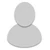## FANDOM

10,619 Pages# B1mb0w

My favorite wikis
•May 18, 2019 by B1mb0w

$$\alpha(5.1713438192857) = Q(t_{0}^{t_{0}^{t_{0}(1)}(Q^{2}(Q^{3}(Q^{t_{0}(0)}(1,t_{0}^{}(0)_*))_*,t_{0}^{}(0)))}(t_{0}(1,0)),3)$$

$$\alpha(5.1713438222485) = Q(t_{0}^{t_{0}^{t_{0}(1)}(Q^{2}(Q(2,t_{0}^{}(0))_*,t_{0}^{}(0)))}(t_{0}(1,0)),3)$$

$$\alpha(5.1713438252114) = Q(t_{0}^{t_{0}^{t_{0}(1)}(Q^{2}(Q^{2}(2,t_{0}^{}(0)_*)_*,t_{0}^{}(0)))}(t_{0}(1,0)),3)$$

$$\alpha(5.1713438281742) = Q(t_{0}^{t_{0}^{t_{0}(1)}(Q^{2}(Q^{t_{0}(0)}(2,t_{0}^{}(0)_*)_*,t_{0}^{}(0)))}(t_{0}(1,0)),3)$$

$$\alpha(5.171343831137) = Q(t_{0}^{t_{0}^{t_{0}(1)}(Q^{2}(Q^{t_{0}(0)}(1,Q^{t_{0}(0)}(2,t_{0}^{}(0)_*)_*)_*,t_{0}^{}(0)))}(t_{0}(1,0)),3)$$

$$\alpha(5.1713438340999) = Q(Q(t_{0}(1,0)),3)$$

$$\alpha(5.17133627) = Q(t_{0… Read more > •## Recursion and Summation Functions March 25, 2019 by B1mb0w I use short hand notation for recursion of nested functions. They are a further development of notation I use that is not in common use. Here is a summary review of my notation: Decremented Function \(C$$ is short-hand notation. For any arbitrary function:

$$M^{c + 1}(n)$$ then $$C = M^{c}(n)$$

e.g.

$$M^{c + 1}(n) = M(C) = M(M^c(n))$$

Parameter Subscript Brackets is shorthand for functions with multiple parameters:

$$M(x,0_{}) = M(x,0,0)$$

$$M(x,y_{}) = M(x,y_1,y_2)$$

$$M(x,0_{},y_{},z) = M(x,0,0,y_1,y_2,y_3,z)$$

Leading Zeros Assumption applies to any function I define which accepts a variable number of input parameters. All leading zeroes can then be ignored:

$$M(0_{[x]},0_{},y_{},1) = M(0_{[x + 2]},y_{},1) = t_0(y_{},1) = M(y_… Read more > •## Alpha Spreadsheet q3.0 February 7, 2019 by B1mb0w Javascript code for my Alpha Function based on my Quantum Function is available in Google Sheets. You are free to use and copy the code as you like. Version 3.0 of the Javascript code in Google Sheets is available here: Alpha Function Spreadsheet This replaces the Version 2.0 spreadsheet. Some examples taken from the spreadsheet. \(\alpha(2) = Q(1,2) = 5$$

$$\alpha(2.75) = Q^{2}(1,2_*) = 11$$

$$\alpha(3.1) = Q^{Q(2)}(1,2_*) = 23$$

$$\alpha(3.61) = Q^{Q^{2}(2)}(1,2_*) = 47$$

$$\alpha(4.29) = Q(t_0(0),2) = 95$$

$$\alpha(4.446) = Q(Q^{t_0(0)}(t_0(0)),2)$$

$$\alpha(4.605) = Q(Q(1,t_0(0)),2)$$

$$\alpha(4.9706) = Q^{Q(Q(1,2))}(Q(Q(t_0(0),t_0(0)),2))$$

$$\alpha(5.8721) = Q(t_0(1),2)$$

$$\alpha(6.143) = Q(Q(t_0(0),t_0(1)),2)$$

$$\alpha(6.436) = Q(Q(t_0(1),t_0(1)… Read more > •## Alpha Function Code q2.0 January 10, 2019 by B1mb0w Javascript code for my Alpha Function based on my Quantum Function is available in Google Sheets. You are free to use and copy the code as you like. Version 2.0 of the Javascript code in Google Sheets is available here: Alpha Function Javascript This version replaces my previous Version 1.0 spreadsheet, but all versions are still available online. Some examples taken from the spreadsheet. \(\alpha(2) = Q(1,2) = 5$$

$$\alpha(2.75) = Q^{2}(1,2_*) = 11$$

$$\alpha(3.1) = Q^{Q(2)}(1,2_*) = 23$$

$$\alpha(3.61) = Q^{Q^{2}(2)}(1,2_*) = 47$$

$$\alpha(4.29) = Q(t_0(0),2) = 95$$

$$\alpha(5.8721) = Q(Q(1,t_0(0)),2)$$

$$\alpha(8.825) = Q(Q(t_0(0),t_0(0)),2)$$

$$\alpha(11.3623) = Q(Q(Q^{t_0(0)}(t_0(0)),t_0(0)),2)$$

$$\alpha(15.2982) = Q(Q(Q(1,t_0(0)),t_0(0)),2)$$

$$\… Read more > •## Alpha Function Code q1.0 December 8, 2018 by B1mb0w Javascript code for my Alpha Function based on my Quantum Function is available in Google Sheets. You are free to use and copy the code as you like. Version 1.0 of the Javascript code in Google Sheets is available here: Alpha Function Javascript Some examples taken from the spreadsheet. \(\alpha(0) = 0$$

$$\alpha(1) = 1$$

$$\alpha(1.375) = 2$$

$$\alpha(1.5) = Q(2) = 3$$

$$\alpha(2) = Q(1,2) = 5$$

$$\alpha(3.01) = Q^{Q^{2}(2)}(Q^{2}(1,2_*)) = Q^{4}(Q^{2}(1,2_*))$$

$$\alpha(4.286) = Q(t_0(0),2)$$

$$\alpha(4.3582) = Q^{Q^{Q^{2}(2)}(1,2_*)}(t_0(0),2_*)$$

$$\alpha(4.3625) = Q(Q(t_0(0)),2)$$

$$\alpha(4.44) = Q(Q^{t_0(0)}(t_0(0)),2)$$

$$\alpha(4.605) = Q(Q(1,t_0(0)),2)$$

$$\alpha(4.9695) = Q(Q(t_0(0),t_0(0)),2)$$

$$\alpha(5.0687) = Q(Q(Q(t_0(0)),t_0(0)),2)$$

\(\a…